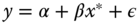# Chapter 6Endogeneity

## 6.1 Introduction

There is an endogeneity problem when the error is correlated with at least one explanatory variable. This phenomenon is very common in econometrics because, compared to experimental sciences, it is not possible (or it is at least difficult) to control the data‐generating process. Among the possible causes of endogeneity, the three most important are:

• simultaneity. In this case, there is an explanatory variable that is set simultaneously with the response: this is, for example, the case when one seeks to estimate a demand equation for a good, which contains the price of the good itself. The demand and the price are simultaneously set by the condition of equality of supply and demand and, therefore, a variation of the error term of the demand equation will shift the demand curve and therefore induce a variation of the quantity and the equilibrium price. The price variable is therefore endogenous.
• covariate measured with error. If the “true” model isand what is observed is, the estimated model writes:, orwith . Hence, is correlated with , which ...

Get Panel Data Econometrics with R now with the O’Reilly learning platform.

O’Reilly members experience books, live events, courses curated by job role, and more from O’Reilly and nearly 200 top publishers.